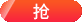Java程序异常处理之try…catch和finally[黑马程序员]

try{
//程序代码块
} catch(ExceptionType (Exception 类及其子类) e) {
//对 ExceptionType的处理
}public class Example{
public static void main(String [] args){
//下面的代码定义了一个 try catch语句用于捕获异常
try{
int result= divide(4,0);    //调用 divide()方法
System.out.println(result);
} catch (Exception e) {     //对异常进行处理
System.out.println("捕获的异常信息为:"+e.getMessage());
}
System.out.printIn("程序继续向下执行……”);
}
//下面的方法实现了两个整数相除
public static divide (int x,int y) {
int result=X / y;    //定义一个变量result记录两个数相乘的结果
return result;      //将结果返回
}
}

D:\cn\itcast\chapter04>java Example

public class Example{
public static void main (String[] args) {
//下面的代码定义了一个try… catch…fina1ly语句用于抽获异常
try {
int result= divide(4，0);  //调用 divide()方法
System.out.println(result);
} catch (Exception e){        //对捕获到的异常进行处理
System.out.println(“捕获的异常信息为:”+e.getMessage());
return;     //用于结束当前语句
} finally {
System.out.println("进人finally代码块”);
}
System.out.println("程序继续向下执行……”);
}
//下面的方法实现了两个整数相除
public static int divide (int x, int y){
int result=x / y; //定义一个变量result记录两个数相除的结果
return result;   //将结果返回
}
}

D:\cn\itcast\chapter04>java Example

相关阅读